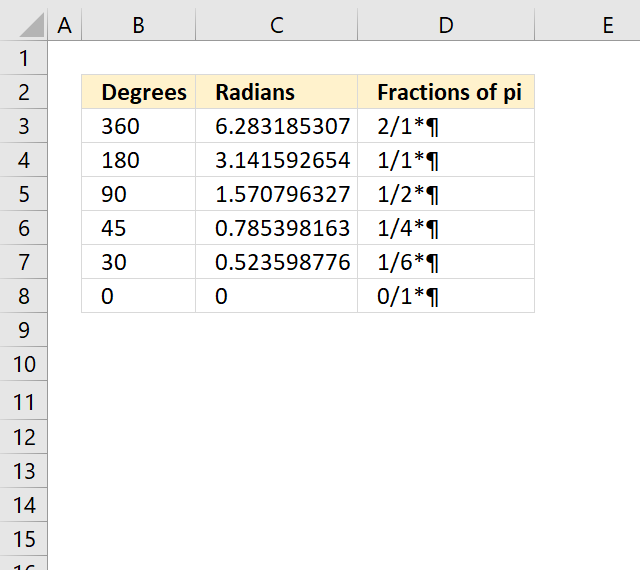Author: Oscar Cronquist Article last updated on March 19, 2018The formula in cell D3 converts the radian decimal value to fractions of pi.

Formula in cell D3:

=TEXT(C3/PI(),"?/?")&"*"&CHAR(182)

### Explaining formula in cell D3

The first operation is the division between the radian number and pi.

C3/PI()

The TEXT function then calculates the fraction.

TEXT(C3/PI(),"?/?")

The CHAR function inserts the pi symbol using ANSI code 182.

CHAR(182)

Lastly, the ampersands concatenate the values.

TEXT(C3/PI(),"?/?")&"*"&CHAR(182)

Convert radians to fractions of pi.xlsx

How to use the RADIANS function